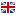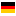# volt ne demek?

1. Elektromotor gücün veya gerilimin birimi.
2. Bir elektrik akımındaki elektromotor gücü gösteren ölçü birimi.
3.Circular tread; a gait by which a horse going sideways round a center makes two concentric tracks.
4.Sudden movement to avoid a thrust.
5.The unit of electrical potential One volt is the electrical potential that will cause one ampere of current to flow through one ohm of resistance.
6.Unit of electrical pressure One volt is the amount of pressure that will cause one ampere of current in one ohm of resistance.
7.The derived SI unit of electric potential difference.
8.The meter-kilogram-second unit of electromotive force, or potential difference; the potential difference between two points in an electric field such that one JOULE of work moves a charge of one COULOMB between these points The electrical force that, if steadily applied to a circuit with a resistance of one OHM, will produce a current of one ampere.
9.Unit of measure for electrical potential.
10.VOLT is a unit of electrical pressure measuring the force or push of electricity.
11.The standard unit of electromotive force or electrical pressure One volt is the amount of pressure that will cause one ampere of current to flow through one ohm of resistance.
12.The quantitative unit of measurement of electrical voltage.
13.Unit of potential difference or electromotive force One volt is the potential difference needed to produce one ampere of current through a resistance of one ohm Voltage is the driving force throughout a sound system from the microphone input to the speaker terminals.
14.Unit of electrical pressure It measures the force or push of electricity Volts represent pressure, correspondent to the pressure of water in a pipe A volt is the unit of electromotive force or electric pressure analogous to water pressure in pounds per square inch It is the electromotive force which, if steadily applied to a circuit having a resistance of one ohm, will produce a current one ampere.
15.The unit of potential difference; joules per coulomb Electrical 'pressure.
16.The unit of electrical potential.
17.Unit of electromotive force.
18.Volt.
19.The unit of measurement of electromotive force necessary to produce one ampere of current in a circuit having a total resistance of one ohm The volt is named for Alessandro Volta, an 18th century Italian physicist.
20.Measure of electrical potential, 110-volt house electricity has more potential to do work than an equal flow of 12-volt electricity.
21.The unit of voltage or potential difference.
22.Unit of measurement for electrical pressure; named after the Italian scientist Alessandro Volta.
23.Unit of electromotive force Amount of force required to drive a steady current of one ampere through a resistance of one ohm current of one.
24.The unit of electrical potential defined as one joule of work per coulomb of charge transferred.
25.The unit of measure for voltage Voltage is the electrical pressure which forces the current to flow in a conductor such as a wire Volt-Ampere Voltage multiplied by the current ; apparent power For instance, a device rated at 10 amps and 120 V has a VA rating of 1200 or 1 2 kVA.
26.Unit that measures the amount of electrical pressure.
27.Unit of potential equal to the potential difference between two points on a conductor carrying a current of 1 ampere when the power dissipated between the two points is 1 watt; equivalent to the potential difference across a resistance of 1 ohm when 1 ampere of current flows through it.
28.Volt
29.Volt
30. Volt, sakınmak için sıçrama, atın bir merkez etrafında yan gitmesi
31. Volt
32. Atın bir merkez etrafında yan yan yürüyerek dolaşması
33. Eskrimde vuruştan sakınmak için yapılan ani sıçrama.

1. Voltamper

### volt amps reactive

1. Var

#### Türetilmiş Kelimeler (bis)

Yorumunuzu ve bilginizi paylaşın